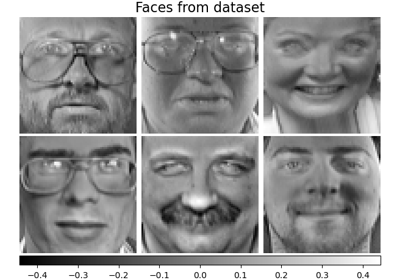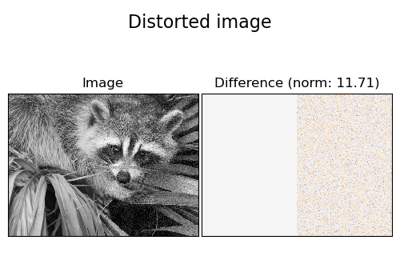# sklearn.decomposition.MiniBatchDictionaryLearning¶

class sklearn.decomposition.MiniBatchDictionaryLearning(n_components=None, *, alpha=1, n_iter='deprecated', max_iter=None, fit_algorithm='lars', n_jobs=None, batch_size=256, shuffle=True, dict_init=None, transform_algorithm='omp', transform_n_nonzero_coefs=None, transform_alpha=None, verbose=False, split_sign=False, random_state=None, positive_code=False, positive_dict=False, transform_max_iter=1000, callback=None, tol=0.001, max_no_improvement=10)[source]

Mini-batch dictionary learning.

Finds a dictionary (a set of atoms) that performs well at sparsely encoding the fitted data.

Solves the optimization problem:

(U^*,V^*) = argmin 0.5 || X - U V ||_Fro^2 + alpha * || U ||_1,1
(U,V)
with || V_k ||_2 <= 1 for all  0 <= k < n_components


||.||_Fro stands for the Frobenius norm and ||.||_1,1 stands for the entry-wise matrix norm which is the sum of the absolute values of all the entries in the matrix.

Read more in the User Guide.

Parameters:
n_componentsint, default=None

Number of dictionary elements to extract.

alphafloat, default=1

Sparsity controlling parameter.

n_iterint, default=1000

Total number of iterations over data batches to perform.

Deprecated since version 1.1: n_iter is deprecated in 1.1 and will be removed in 1.4. Use max_iter instead.

max_iterint, default=None

Maximum number of iterations over the complete dataset before stopping independently of any early stopping criterion heuristics. If max_iter is not None, n_iter is ignored.

New in version 1.1.

fit_algorithm{‘lars’, ‘cd’}, default=’lars’

The algorithm used:

• 'lars': uses the least angle regression method to solve the lasso problem (linear_model.lars_path)

• 'cd': uses the coordinate descent method to compute the Lasso solution (linear_model.Lasso). Lars will be faster if the estimated components are sparse.

n_jobsint, default=None

Number of parallel jobs to run. None means 1 unless in a joblib.parallel_backend context. -1 means using all processors. See Glossary for more details.

batch_sizeint, default=256

Number of samples in each mini-batch.

Changed in version 1.3: The default value of batch_size changed from 3 to 256 in version 1.3.

shufflebool, default=True

Whether to shuffle the samples before forming batches.

dict_initndarray of shape (n_components, n_features), default=None

Initial value of the dictionary for warm restart scenarios.

transform_algorithm{‘lasso_lars’, ‘lasso_cd’, ‘lars’, ‘omp’, ‘threshold’}, default=’omp’

Algorithm used to transform the data:

• 'lars': uses the least angle regression method (linear_model.lars_path);

• 'lasso_lars': uses Lars to compute the Lasso solution.

• 'lasso_cd': uses the coordinate descent method to compute the Lasso solution (linear_model.Lasso). 'lasso_lars' will be faster if the estimated components are sparse.

• 'omp': uses orthogonal matching pursuit to estimate the sparse solution.

• 'threshold': squashes to zero all coefficients less than alpha from the projection dictionary * X'.

transform_n_nonzero_coefsint, default=None

Number of nonzero coefficients to target in each column of the solution. This is only used by algorithm='lars' and algorithm='omp'. If None, then transform_n_nonzero_coefs=int(n_features / 10).

transform_alphafloat, default=None

If algorithm='lasso_lars' or algorithm='lasso_cd', alpha is the penalty applied to the L1 norm. If algorithm='threshold', alpha is the absolute value of the threshold below which coefficients will be squashed to zero. If None, defaults to alpha.

Changed in version 1.2: When None, default value changed from 1.0 to alpha.

verbosebool or int, default=False

To control the verbosity of the procedure.

split_signbool, default=False

Whether to split the sparse feature vector into the concatenation of its negative part and its positive part. This can improve the performance of downstream classifiers.

random_stateint, RandomState instance or None, default=None

Used for initializing the dictionary when dict_init is not specified, randomly shuffling the data when shuffle is set to True, and updating the dictionary. Pass an int for reproducible results across multiple function calls. See Glossary.

positive_codebool, default=False

Whether to enforce positivity when finding the code.

New in version 0.20.

positive_dictbool, default=False

Whether to enforce positivity when finding the dictionary.

New in version 0.20.

transform_max_iterint, default=1000

Maximum number of iterations to perform if algorithm='lasso_cd' or 'lasso_lars'.

New in version 0.22.

callbackcallable, default=None

A callable that gets invoked at the end of each iteration.

New in version 1.1.

tolfloat, default=1e-3

Control early stopping based on the norm of the differences in the dictionary between 2 steps. Used only if max_iter is not None.

To disable early stopping based on changes in the dictionary, set tol to 0.0.

New in version 1.1.

max_no_improvementint, default=10

Control early stopping based on the consecutive number of mini batches that does not yield an improvement on the smoothed cost function. Used only if max_iter is not None.

To disable convergence detection based on cost function, set max_no_improvement to None.

New in version 1.1.

Attributes:
components_ndarray of shape (n_components, n_features)

Components extracted from the data.

n_features_in_int

Number of features seen during fit.

New in version 0.24.

feature_names_in_ndarray of shape (n_features_in_,)

Names of features seen during fit. Defined only when X has feature names that are all strings.

New in version 1.0.

n_iter_int

Number of iterations over the full dataset.

n_steps_int

Number of mini-batches processed.

New in version 1.1.

DictionaryLearning

Find a dictionary that sparsely encodes data.

MiniBatchSparsePCA

Mini-batch Sparse Principal Components Analysis.

SparseCoder

Find a sparse representation of data from a fixed, precomputed dictionary.

SparsePCA

Sparse Principal Components Analysis.

References

J. Mairal, F. Bach, J. Ponce, G. Sapiro, 2009: Online dictionary learning for sparse coding (https://www.di.ens.fr/sierra/pdfs/icml09.pdf)

Examples

>>> import numpy as np
>>> from sklearn.datasets import make_sparse_coded_signal
>>> from sklearn.decomposition import MiniBatchDictionaryLearning
>>> X, dictionary, code = make_sparse_coded_signal(
...     n_samples=30, n_components=15, n_features=20, n_nonzero_coefs=10,
...     random_state=42)
>>> dict_learner = MiniBatchDictionaryLearning(
...     n_components=15, batch_size=3, transform_algorithm='lasso_lars',
...     transform_alpha=0.1, max_iter=20, random_state=42)
>>> X_transformed = dict_learner.fit_transform(X)


We can check the level of sparsity of X_transformed:

>>> np.mean(X_transformed == 0) > 0.5
True


We can compare the average squared euclidean norm of the reconstruction error of the sparse coded signal relative to the squared euclidean norm of the original signal:

>>> X_hat = X_transformed @ dict_learner.components_
>>> np.mean(np.sum((X_hat - X) ** 2, axis=1) / np.sum(X ** 2, axis=1))
0.052...


Methods

 fit(X[, y]) Fit the model from data in X. fit_transform(X[, y]) Fit to data, then transform it. get_feature_names_out([input_features]) Get output feature names for transformation. Get metadata routing of this object. get_params([deep]) Get parameters for this estimator. partial_fit(X[, y]) Update the model using the data in X as a mini-batch. set_output(*[, transform]) Set output container. set_params(**params) Set the parameters of this estimator. Encode the data as a sparse combination of the dictionary atoms.
fit(X, y=None)[source]

Fit the model from data in X.

Parameters:
Xarray-like of shape (n_samples, n_features)

Training vector, where n_samples is the number of samples and n_features is the number of features.

yIgnored

Not used, present for API consistency by convention.

Returns:
selfobject

Returns the instance itself.

fit_transform(X, y=None, **fit_params)[source]

Fit to data, then transform it.

Fits transformer to X and y with optional parameters fit_params and returns a transformed version of X.

Parameters:
Xarray-like of shape (n_samples, n_features)

Input samples.

yarray-like of shape (n_samples,) or (n_samples, n_outputs), default=None

Target values (None for unsupervised transformations).

**fit_paramsdict

Returns:
X_newndarray array of shape (n_samples, n_features_new)

Transformed array.

get_feature_names_out(input_features=None)[source]

Get output feature names for transformation.

The feature names out will prefixed by the lowercased class name. For example, if the transformer outputs 3 features, then the feature names out are: ["class_name0", "class_name1", "class_name2"].

Parameters:
input_featuresarray-like of str or None, default=None

Only used to validate feature names with the names seen in fit.

Returns:
feature_names_outndarray of str objects

Transformed feature names.

Get metadata routing of this object.

Please check User Guide on how the routing mechanism works.

Returns:

A MetadataRequest encapsulating routing information.

get_params(deep=True)[source]

Get parameters for this estimator.

Parameters:
deepbool, default=True

If True, will return the parameters for this estimator and contained subobjects that are estimators.

Returns:
paramsdict

Parameter names mapped to their values.

partial_fit(X, y=None)[source]

Update the model using the data in X as a mini-batch.

Parameters:
Xarray-like of shape (n_samples, n_features)

Training vector, where n_samples is the number of samples and n_features is the number of features.

yIgnored

Not used, present for API consistency by convention.

Returns:
selfobject

Return the instance itself.

set_output(*, transform=None)[source]

Set output container.

See Introducing the set_output API for an example on how to use the API.

Parameters:
transform{“default”, “pandas”}, default=None

Configure output of transform and fit_transform.

• "default": Default output format of a transformer

• "pandas": DataFrame output

• None: Transform configuration is unchanged

Returns:
selfestimator instance

Estimator instance.

set_params(**params)[source]

Set the parameters of this estimator.

The method works on simple estimators as well as on nested objects (such as Pipeline). The latter have parameters of the form <component>__<parameter> so that it’s possible to update each component of a nested object.

Parameters:
**paramsdict

Estimator parameters.

Returns:
selfestimator instance

Estimator instance.

transform(X)[source]

Encode the data as a sparse combination of the dictionary atoms.

Coding method is determined by the object parameter transform_algorithm.

Parameters:
Xndarray of shape (n_samples, n_features)

Test data to be transformed, must have the same number of features as the data used to train the model.

Returns:
X_newndarray of shape (n_samples, n_components)

Transformed data.

## Examples using sklearn.decomposition.MiniBatchDictionaryLearning¶Faces dataset decompositions

Faces dataset decompositionsImage denoising using dictionary learning

Image denoising using dictionary learning